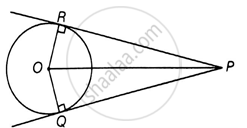# Two tangents PQ and PR are drawn from an external point to a circle with centre O. Prove that QORP is a cyclic quadrilateral. - Mathematics

Diagram
Sum

Two tangents PQ and PR are drawn from an external point to a circle with centre O. Prove that QORP is a cyclic quadrilateral.

#### SolutionGiven: PQ and PR are two tangents drawn at points Q and R are drawn from an external point P.

To Prove: QORP is a cyclic Quadrilateral.

Proof: OR ⏊ PR and OQ ⏊PQ   ......[Tangent at a point on the circle is perpendicular to the radius through point of contact]

∠ORP = 90°

∠OQP = 90°

∠ORP + ∠OQP = 180°

Hence QOPR is a cyclic quadrilateral. As the sum of the opposite pairs of angle is 180°

Concept: Concept of Circle - Centre, Radius, Diameter, Arc, Sector, Chord, Segment, Semicircle, Circumference, Interior and Exterior, Concentric Circles
Is there an error in this question or solution?

#### APPEARS IN

NCERT Mathematics Exemplar Class 10
Chapter 9 Circles
Exercise 9.3 | Q 2 | Page 107
Share